Electron. J. Diff. Equ., Vol. 2012 (2012), No. 85, pp. 1-36.

### Existence and concentration of semiclassical states for nonlinear Schrodinger equations Shaowei Chen

Abstract: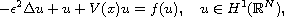where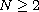and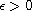is a small parameter. The function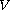is bounded in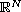,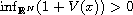and it has a possibly degenerate isolated critical point. Under some conditions on f, we prove that as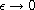, this equation has a solution which concentrates at the critical point of V.Shaowei Chen School of Mathematical Sciences Capital Normal University Beijing 100048, China email: chensw@amss.ac.cn